### Galaxy Watch

### Tag Expression

Use tag expressions to create unique and awesome watch faces that change dynamically.Note Tag expressions are not supported by Samsung Gear Fit watches.

Tag expressions are conditions that allow you to change the rotation, placement, and opacity of a component based on tag values (watch data) such as date and time, battery status, caffeine or water intake, calories burned, steps, or heart rate. Your watch face changes dynamically as the tag value changes. For example, you can create a watch face where the object on the screen moves vertically or horizontally every second, or you can create a watch face with objects that blink at certain times.

Watch data is represented by tag IDs or tags whose range of values is shown in the following tables:

Data Tag ID Range
Hour in day (0-23) [H] 0 - 23
Minute in Hour [m] 0 - 59
Second in Minute [s] 0 - 59
Day of Month [d] 1 - 31
Month of Year [M] 1 - 12
Day of Week [e] 1 - 7
Day of Year [D] 0.0 - 11.99
Data Tag ID Range
Battery (%) [ba] 0 - 100
Battery charging status (0/1) [bc] 0/1
Battery Level [bl] 0 – 4
Steps (%) [st] 0 - 100
Step counts [sc] 0 - ∞
Steps goal [sg] 0 - ∞
Speed (m/s) [sp] 0 - ∞
Burned calorie (cal) [cal] 0 - ∞
Moved distance (Km/h) [md] 0 - ∞
Heart rate (bpm) [hr] 0 - ∞
Water intake [wi] 0 - ∞
Caffeine intake [ci] 0 - ∞
Floor [fl] 0 - ∞
Moon phase position [mp] 0 – 28

You can enter a tag expression directly into the Rotate, Placement, and Opacity properties input fields of a component. Tags must be enclosed in square brackets [ ]. Input fields that allow tag expressions have a triangular icon in the lower left corner.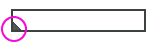To open a larger input window to enter complex or long formulas, click the triangle icon or double-click the input field. In the Script window, you can enter complex and lengthy tag expressions without truncation, and you can easily search for the tags for the data you want.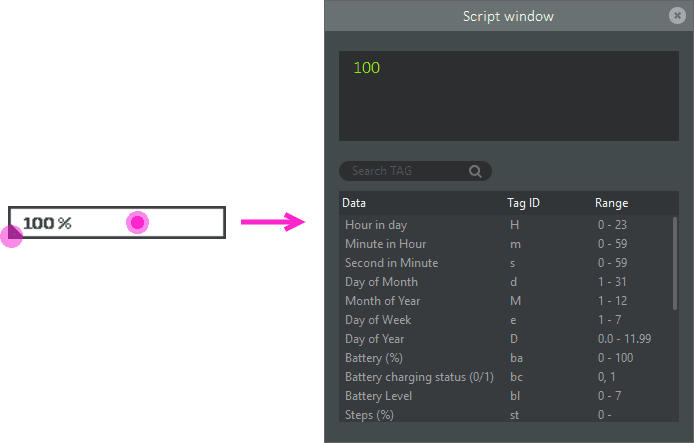Arithmetic operators

Arithmetic operators are basic math functions. They perform a function between two values (operands).

Arithmetic operator Function
- Subtraction
* Multiplication
/ Quotient of division
% Remainder of division

The precedence of arithmetic operations follows the same precedence as mathematic operations:

1. 1.

Operations inside parentheses are performed first.

2. 2.

Multiplication and division arithmetic operations are performed next. If there are multiple multiplication and division operations in an expression, they are performed in order, from left to right.

3. 3.

Finally, addition and subtraction arithmetic operations are performed. If there are multiple addition and subtraction operations in an expression, they are performed in order, from left to right.

Examples

The following examples show how to use tags and arithmetic operations in a tag expression:

• 5 * [ba] : Returns battery percentage multiplied by 5. If the battery percentage is 7%, then the value returned is 35. That is, 5 * 7 = 35.

• [s] / 3 : Returns the quotient of seconds in a minute divided by 3. If the number of seconds that have passed in the minute is 7, then the value returned is 2.

• [s] % 3 : Returns the remainder of seconds in a minute divided by 3. If the number of seconds that have passed in the minute is 7, then the value returned is 1.

• 3 + 5 * [ba] : Battery percentage is multiplied by 5, then 3 is added. If the battery percentage is 7%, then the value returned is 38. Multiplication is performed first (because it takes precedence over addition) and then 3 is added to that result: 3 + 5 * 7 → 3 + 35 → 38.

• (3 + 5) * [ba] : 3 is added to 5 first, then the result is multiplied by the battery percentage. If the battery percentage is 7%, then the value returned is 56. The addition operation in parentheses is performed first then the result is multiplied by the battery percentage: (3 + 5) * 7 → 8 * 7 → 56.

Relational operators

Relational operators compare two values to determine which is greater, less than, or equal to each other. A comparison that is true returns a value of 1. A comparison that is false returns a value of 0 (zero).

Relational operator Function
< Less than
<= Less than or equal to
> Greater than
>= Greater than or equal to
== Equal to
!= Not equal to

Examples

Relational operations are sometimes combined with arithmetic operations. Here is an example that uses only a relational operation and another example that uses both a relational operation and arithmetic operations.

• [ba] <= 15

• If the battery percentage is less than or equal to 15%, [ba] <= 15 is true and returns the value of 1.
• If the battery percentage is greater than 15%, [ba] <= 15 is false and returns the value of 0 (zero).
• 1000 * ([hr] < 100) + 120

• The operation in parentheses, the relational operation, must be performed first.
• If the heart rate is less than 100 bpm, [hr] < 100 is true and returns the value of 1. Next, multiply 1 by 1,000 and then add 120. The final value returned is 1,120.
For example, if [hr] = 80 bpm: 1000 * ([hr] < 100) + 120 → 1000 * (80 < 100) + 120 → 1000 * (1) + 120 → 1,120.
• If the heart rate is greater than or equal to 100 bpm, [hr] < 100 is false and returns the value of 0 (zero). Next, multiply 0 by 1,000 and then add 120. The final value returned is 120.
For example, if [hr] = 120 bpm: 1000 * ([hr] < 100) + 120 → 1000 * (120 < 100) + 120 → 1000 * (0) + 120 → 120.

Creating a pedometer (using relational operations)

Using relational operations, let’s create a simple pedometer. The pedometer displays one of two images, depending on the step percentage (the [st] tag). If [st] is 0%, the watch displays the image of a man standing still. If [st] is 100%, the watch displays the image of a man raising his arms in victory. If [st] is between 0% and 100%, nothing is displayed. To display an image, set its opacity to 100. To hide an image, set its opacity to 0.

• Display the standing man image if step percentage is equal to 0%.Set Opacity to ([st] == 0) * 100.

The operation in parentheses, a relational operation, must be resolved first. When [st] is equal to 0%, ([st] == 0) returns 1. The standing man image is displayed because the Opacity property is set to 100. When [st] is not equal to 0%, ([st] == 0) returns 0. The standing man image is not displayed because the Opacity property is set to 0.

If [st] = 0,

• The relational operation ([st] == 0) returns 1 (true)

• Opacity = (0 == 0) * 100 → (1 ) * 100 → 100

• Opacity is set to 100 and the standing man image is displayed

If [st] > 0,

• The relational operation ([st] == 0) returns 0 (false)

• Opacity = ([st] == 0) * 100 → (0) * 100 → 0

• Opacity is set to 0 and the standing man image is not displayed

Let’s walk through the calculation of the tag expression ([st] == 0) * 100 when step percentage is 0.

• Replace [st] with the value, in this case 0 (zero):([st] == 0) * 100 → (0 == 0) * 100

• The operation in parentheses must be performed first, so perform the relational operation in parentheses (0 == 0). Because 0 is equal to 0, the relational operation returns 1 (true): (0 == 0) * 100 → (1) * 100

• Now, calculate 1 multiplied by 100 which results in 100. Note that * is interpreted as an arithmetic operation because only one of its operands is a relational operation ([st] == 0) while the other is a value (100): (1) * 100 → 100

• Display the victorious man image if step percentage is equal to 100%.Set Opacity to ([st] == 100) * 100.

The operation in parentheses, a relational operation, must be resolved first. When [st] is equal to 100%, ([st] == 100) returns 1. The victorious man image is displayed because the Opacity property is set to 100. When [st] is not equal to 100%, ([st] == 100) returns 0. The victorious man image is not displayed because the Opacity property is set to 0.

If [st] < 100,

• The relational operation ([st]) == 100) returns 0 (false)

• Opacity = ([st] == 100) * 100 → (0) * 100 → 0

• Opacity is set to 0 and the victorious man image is not displayed

If [st] = 100,

• The relational operation ([st]) == 100) returns 1 (true)

• Opacity = (100 == 100) * 100 → (1) * 100 → 100

• Opacity is set to 100 and the victorious man image is displayed

Let’s walk through the calculation of the tag expression ([st] == 100)*100 when step percentage is 0.

• Replace [st] with the value, in this case 0:([st] == 100) * 100 → (0 == 100) * 100

• The operation in parentheses must be resolved first, so resolve the relational operation in parentheses (0 == 100). Because 0 does not equal 100, the relational operation returns 0 (false): (100 == 100) * 100 → (0) * 100

• Now, calculate 0 multiplied by 100 which results in 0. Note that * is interpreted as an arithmetic operation because only one of its operands is a relational operation ([st] == 100) while the other is a value (100): (0) * 100 → 0

Logical operators

Logical operators combine the results of two or more relational operations and returns a value of 1 (true) or 0 (false). Tag expressions support two types of logical operations: AND and OR.

• AND – Represented by an asterisk (*). Both relational operations must be true in order for the AND logical operator to return a value of 1 (true). Otherwise, the return value is 0 (false). Note that an asterisk also represents the multiplication arithmetic operator.

• OR – Represented by the plus sign (+). One or more of the relational operations must be true in order for the OR logical operator to return a value of 1 (true). Only if both of the relational operations are false, will the return value be 0 (false). Note that the plus sign also represents the addition arithmetic operator.

Here is a summary of the results of a logical operation:

Result of relational operation 1 Result of relational operation 2 Result of AND (*)
(relational operation 1) * (relational operation 2)
Result of OR (+)
(relational operation 1) + (relational operation 2)
0 (false) 0 (false) 0 * 0 = 0 0 + 0 = 0
0 (false) 1 (true) 0 * 1 = 0 0 + 1 = 1
1 (true) 0 (false) 1 * 0 = 0 1 + 0 = 1
1 (true) 1 (true) 1 * 1 = 1 1 + 1 = 1

How does the tag expression know when to use a logical or arithmetic operator? A logical operator is applied only if both of the two operands (the values on either side of the operator) are relational operations. An arithmetic operator is applied if either of the two operands is a number or value.

Logical operation Arithmetic operation Arithmetic operation
Both operands are relational operations:
(0 <= 1) * (1 < 2)
Result: 1
One operand is a relational operation and the other is a value:
(0 <= 1) *120
Result: 120
Both operands are values:
1*120
Result: 120
This tag expression is made up of two relational operations on either side of the operator (0<=1 and 1<2). Because of this, the logical operator is applied. Since both of the relational operations are true, the final result of this tag expression is 1 (true). This tag expression is made up of a relational operation (0<=1) and a value (120). Because of this, the arithmetic operator is applied. The result of the relational operation is 1 (true) which is then multiplied by 120. The final result of this operation is 120. This tag expression is made up of two values (1 and 120). Because of this, the arithmetic operator is applied. The result is 1 multiplied by 120 which returns a final result of 120.

Examples

Here are two examples using logical operations combined with arithmetic operations.

• 100 * (([H] >= 6) * ([H] <= 17))

Determine if the hour in the day is greater than or equal to 6 AND less than or equal to 17, multiply the result by 100.

• If [H] =3, then the final resulting value is 0.
• First, compute the relational operations: ([H] >= 6) returns 0 (false) and ([H] <= 17) returns 1 (true)
• Next, compute the logical operation: (([H] >= 6) * ([H] <= 17)) → ((0) * (1)) → 0 AND 1 → 0
• Finally, compute the arithmetic operation: 100 * 0 → 0
• If [H] = 9, then the final resulting value is 100.
• First, compute the relational operations: ([H] >= 6) returns 1 (true) and ([H] <= 17) returns 1 (true)
• Next, compute the logical operation: (([H] >= 6) * ([H] <= 17)) → (1) * (1) → 1 AND 1 → 1
• Finally, compute the arithmetic operation: 100 * 1 → 100
• If [H] = 20, then the final resulting value is 0.
• First, compute the relational operations: ([H] >= 6) returns 1 (true) and ([H] <= 17) returns 0 (false)
• Next, compute the logical operation: (([H] >= 6) * ([H] <= 17)) → (1) * (0) → 1 AND 0 → 0
• Finally, compute the arithmetic operation: 100 * 0 → 0
• 120 + (([hr] <= 50) + ([hr] >= 100))

Determine if your heart rate is less than or equal to 50 bpm OR greater than or equal to 100 bpm, add 120 to the result.

• If [hr] = 30, then the resulting value is 121.
• First, compute the relational operations: ([hr] <= 50) returns 1 (true) and ([hr] >= 100) returns 0 (false)
• Next, compute the logical operation: (([hr] <= 50) + ([hr] >= 100)) → (1) + (0) → 1 OR 0 → 1
• Finally, compute the arithmetic operation: 120 + 1 → 121
• If [hr] = 70, then the resulting value is 120.
• First, compute the relational operations: ([hr] <= 50) returns 0 (false) and ([hr] >= 100) returns 0 (false)
• Next, compute the logical operation: (([hr] <= 50) + ([hr] >= 100)) → (0) + (0) → 0 OR 0 → 0
• Finally, compute the arithmetic operation: 120 + 0 → 120
• If [hr] = 120, then the resulting value is 121.
• First, compute the relational operations: ([hr] <= 50) returns 0 (false) and ([hr] >= 100) returns 1 (true)
• Next, compute the logical operation: (([hr] <= 50) + ([hr] >= 100)) → (0) + (1) → 0 OR 1 → 1
• Finally, compute the arithmetic operation: 120 + 1 → 121

Creating a pedometer (using logical and relational operations)

Using logical and relational operations, let’s add another image to our pedometer. This pedometer displays not only the standing man when step percentage (the [st] tag) is 0% and the victorious man when step percentage is 100%, but also an image of a walking man if the step percentage is greater than or equal to 1% and less than or equal to 99%.

•Display the standing man image if:
step percentage = 0%

•Display the walking man image if:
1% <= step percentage <= 99%

•Display the victorious man image if:
step percentage = 100%

The standing man and victorious man images

•The tag expression for opacity is the same as the previous pedometer example.

Set Opacity to ([st] == 0) * 100.

•The tag expression for opacity is the same as the previous pedometer example.

Set Opacity to ([st] == 100) * 100.

The walking man image

•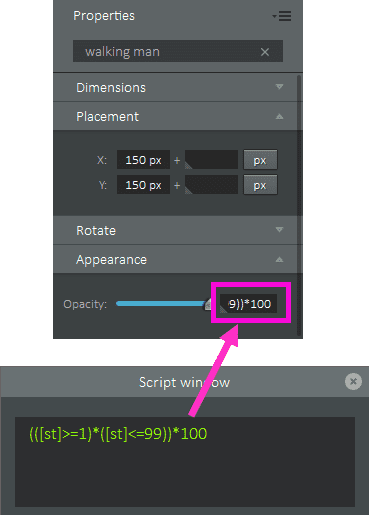• Set Opacity to (([st] >= 1) * ([st] <= 99)) * 100.

When [st] is equal to 0, ([st] >= 1) * ([st] <= 99) returns 0. The walking man image is not displayed because the Opacity property is set to 0.

When [st] is greater than or equal to 1 AND [st] is less than or equal to 99, ([st] >= 1) * ([st] <= 99) returns 1. The walking man image is displayed because the Opacity property is set to 100.

When [st] is equal to 100, ([st] >= 1) * ([st] <= 99) returns 0. The walking man image is not displayed because the Opacity property is set to 0.

If [st] = 0,

• The first relational operation ([st] >= 1) returns 0 (false) and the second relational operation ([st] <= 99) returns 1 (true)

• The logical operation (([st] >= 1)*([st] <= 99)) returns 0 (false)
([st] >= 1) * ([st] <= 99) → (0 >= 1) * (0 <= 99) → (0) * (1) → 0

• Opacity = ((0 >= 1) * (0 <= 99)) * 100 → ((0) * (1)) * 100 → (0) * 100 → 0

• Opacity is set to 0 and the walking man image is not displayed

If [st] >= 1 and [st] <= 99,

• The first relational operation ([st]>=1) returns 1 (true) and the second relational operation ([st] <= 99) returns 1 (true)

• The logical operation (([st] >= 1) * ([st] <= 99)) returns 1 (true)
([st] >= 1) * ([st] <= 99) → (1) * (1) → 1

• Opacity = (([st] >= 1) * ([st] <= 99)) * 100 → ((1) * (1)) * 100 → (1) * 100 → 100

• Opacity is set to 100 and the walking man image is displayed

If [st] = 100,

• The first relational operation ([st]>=1) returns 1 (true) and the second relational operation ([st]<=99) returns 0 (false)

• The logical operation (([st] >= 1) * ([st] <= 99)) returns 0 (false)
([st] >= 1) * ([st] <= 99) → (100 >= 1) * (100 <= 99) → (1) * (0) → 0

• Opacity = ((100 >= 1) * (100 <= 99)) * 100 → ((1) * (0) * 100 → (0) * 100 → 0

• Opacity is set to 0 and the walking man image is not displayed

Let’s walk through the calculation of the tag expression (([st] >= 1) * ([st] <= 99)) * 100 when step percentage is 50.

• Replace [st] with the value, in this case 50: (([st] >= 1) * ([st] <= 99)) * 100 → ((50 >= 1) * (50 <= 99)) * 100

• The operations in parentheses must be resolved first, so resolve the relational operations (50 >= 1) and (50 <= 99). Because 50 is greater than 1, the first relational operation returns 1 (true). Because 50 is less than 99, the second relational operation returns 1 (true): ((50 >= 1) * (50 <= 99)) * 100 → ((1) * (1)) * 100

• Again, the operation in parentheses must be resolved first, so resolve the logical operation ((1) * (1)). In this case, * is interpreted as the AND logical operation because the operands on both sides of the operator are relational operations. (1 AND 1) returns 1 (true): ((1) * (1)) * 100 → (1) * 100

• Now, calculate 1 multiplied by 100 which results in 100. Note that * is interpreted as an arithmetic operation because only one of its operands is a logical operation while the other is a value (100): (1) * 100 → 100

Creating a pedometer (using logical, relational, and arithmetic operations)

Let’s create another pedometer that adjusts the placement of each image, starting with the standing man on the left, the victorious man on the right, and the walking man between the two with its placement changing based on the percentage of steps taken. This example is also part of the Steps_Digital_B complication provided with the Galaxy Watch Designer (Component bar > Complications > Workout > Steps_Digital_B).

•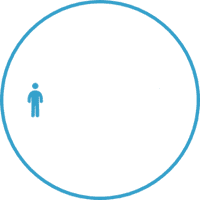Display the standing man image if:
step percentage = 0%

•Display the walking man image if:
1% <= step percentage <= 99% with the placement of the image dependent on the step percentage

•Display the victorious man image if:
step percentage = 100%

The standing man and victorious man images

•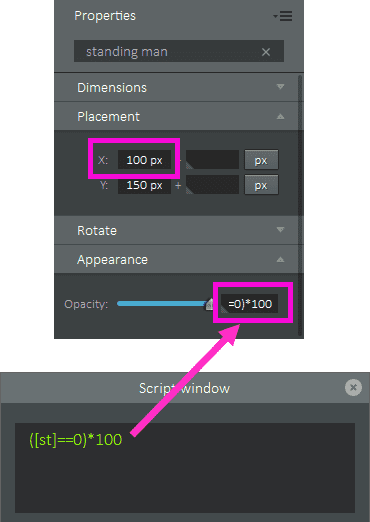Opacity

The tag expression for opacity is the same as the previous pedometer example.

Set Opacity to ([st] == 0) * 100.

Placement

Note that the horizontal (X) value for Placement has been adjusted so that the image appears on the left.

•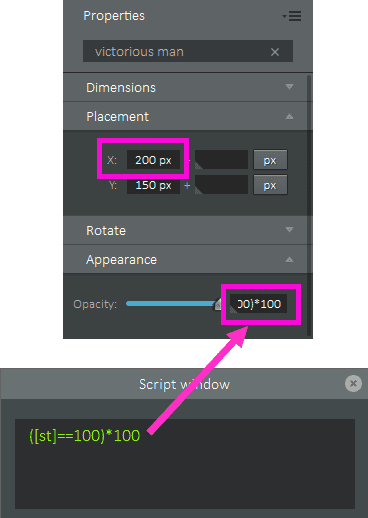Opacity

The tag expression for opacity is the same as the previous pedometer example.

Set Opacity to ([st] == 100) * 100.

Placement

Note that the horizontal (X) value for Placement has been adjusted so that the image appears on the right.

The walking man image

•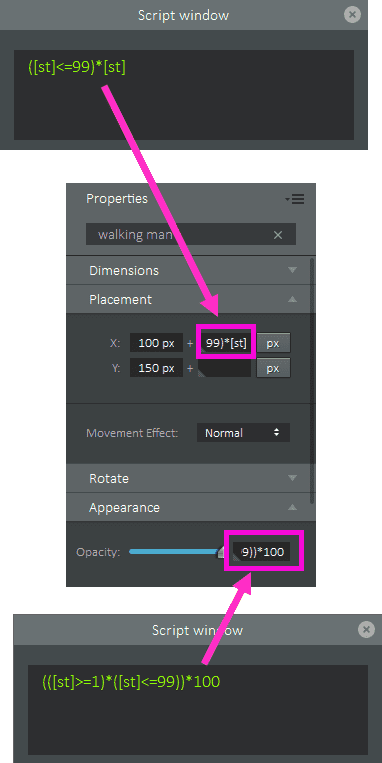• Opacity

The tag expression for opacity is the same as the previous pedometer example

Set Opacity to (([st] >= 1) * ([st] <= 99)) * 100.

Placement

The X Placement property contains a tag expression, ([st] <= 99) * [st]. The operation in parentheses must be resolved first. The operation uses a relational operator and returns 1 (true) or 0 (false), based on the result of the operation. The X Placement property positions the walking man image along the x-axis on the watch face (from 100px to 199px). As the step percentage increases, the tag expression result increases, and the placement of the walking man image moves further to the left of its starting position. That is, for each increase of 1% of step percentage, the walking man image moves to the left by 1px. Because the Y Placement property (y-axis) does not change, the walking man image moves in a horizontal line across the watch face.

If [st] = 0,

• The relational operation ([st] <= 99) returns 1 (true)

• X = 100 + (0 <= 99) * 0 → 100 + (1) * 0 → 100 + 0 → 100

• However, because Opacity is 0 when [st] = 0, the walking man image is not displayed

If [st] = 50,

• The relational operation ([st] <= 99) returns 1 (true)

• X = 100 + (50 <= 99) * 50 → 100 + (1) * 50 → 100 + 50 → 150

If [st] = 100,

• The relational operation ([st] <= 99) returns 0 (false)

• X = 100 + (100 <= 99) * 100 → 100 + (0) * 100 → 100 + 0 → 100

• However, because Opacity is 0 when [st] = 100, the walking man image is not displayed.

Ternary operators

Ternary operations have three operands: a conditional operation, a result if the conditional operation is true, and a result if the conditional operation is false. A conditional operation can be a relational or logical operation. It is presented in the following format:

conditional_operation? result_if_true: result_if_false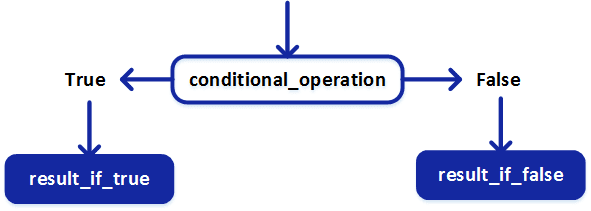Examples

The following examples are used to set the opacity of an image on the watch face.

• [ba] <= 20? 15: 100

Set the opacity of an image on the watch face to 15 if battery percentage is less than or equal to 20. Otherwise, set the opacity to 100.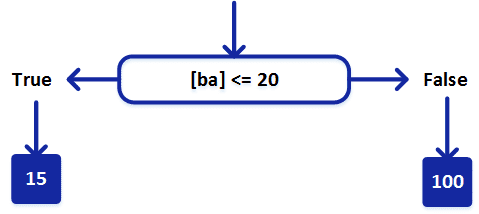• If [ba] = 10,
• [ba] <= 20 returns true
• Opacity is set to 15
• If [ba] = 60,
• [ba] <= 20 returns false
• Opacity is set to 100
• ([ba] >= 50) * ([ba] <= 75)? 100: 0

This example uses a logical operation for its conditional operation. Set the opacity of an image on the watch face to 100 if battery percentage is greater than or equal to 50 and less than or equal to 75. Otherwise, set the opacity to 0.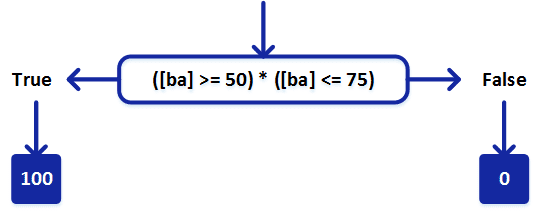• If [ba] = 10,
• First, compute the relational operations: ([ba] >= 50) returns 0 (false) and ([ba] <= 75) returns 1 (true)
• Next, compute the logical operation: ([ba] >= 50) * ([ba] <= 75) → (10 >= 50) * (10 <= 75) → (0) * (1) → 0 AND 1 → 0 (false)
• Finally, since the conditional operation returns false, opacity is set to 0
• If [ba] = 60,
• First, compute the relational operations: ([ba] >= 50) returns true (1) and ([ba] <= 75) returns true (1)
• Next, compute the logical operation: ([ba] >= 50) * ([ba] <= 75) → (60 >= 50) * (60 <= 75) → (1) * (1) → 1 AND 1 → 1 (true)
• Finally, since the conditional operation returns true, opacity is set to 100
• If [ba] = 90,
• First, compute the relational operations: ([ba] >= 50) returns true (1) and ([ba] <= 75) returns false (0)
• Next, compute the logical operation: ([ba] >= 50) * ([ba] <= 75) → (90 >= 50) * (90 <= 75) → (1) * (0) → 1 AND 0 → 0 (false)
• Finally, since the conditional operation returns false, opacity is set to 0

Nested ternary operations

Ternary operations can be nested within the result operand of another ternary operation.

For example, if you have two ternary operations (a? b: c) and (x? y: z), you could create the following nested ternary operations:

• conditional_operation? (a? b: c): result_if_false – If conditional_operation is true, then check conditional operation a. If a is true, the result is b. If a is false, the result is c. If conditional_operation is false, the result is result_if_false.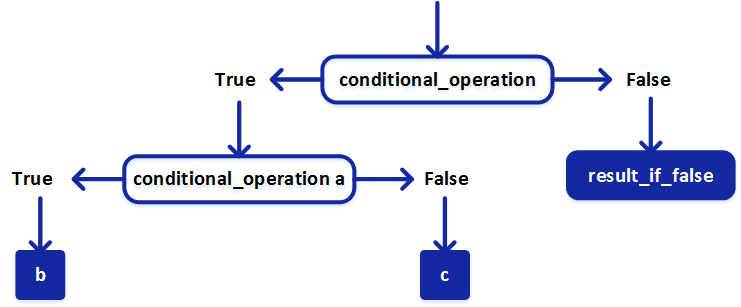• conditional_operation? result_if_true: (x? y: z) – If conditional_operation is true, the result is result_if_true. If conditional_operation is false, then check conditional operation x. If x is true, the result is y. If x is false, the result is z.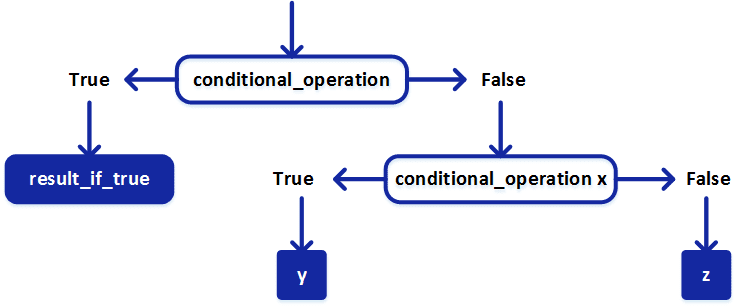• conditional_operation? (a? b: c): (x? y: z) – If conditional_operation is true, then check conditional operation a. If a is true, the result is b. If a is false, the result is c. If conditional_operation is false, then check conditional operation x. If x is true, the result is y. If x is false, the result is z.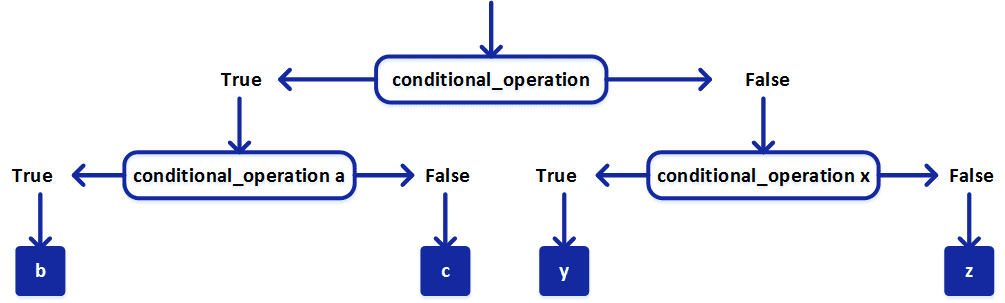Example

The following nested ternary operation is used to set the opacity of an image on the watch face. In this example, the conditional operations are logical operations.

([hr] >= 100) * ([hr] <= 140) ? (([sp] >= 2) * ([sp] <=6 )? 50: 100): 0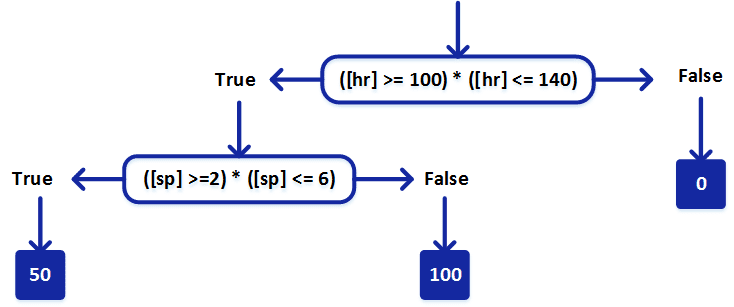Opacity is determined using heart rate ([hr]) and speed ([sp]).

Heart rate: the first conditional operation

The first conditional operation that must be considered is ([hr] >= 100) * ([hr] <= 140): if heart rate is greater than or equal to 100bpm and heart rate is less than or equal to 140bpm. Note that this conditional operation is also a logical operation because its two operands are relational operations.

• If [hr] = 90,

• First, compute the relational operations: ([hr] >= 100) returns 0 (false) and ([hr] <= 140) returns 1 (true)
• Next, compute the logical operation: ([hr] >= 100) * ([hr] <= 140) → (90 >= 100) * (90 <= 140) → (0) * (1) → 0 (false)
• Finally, since the conditional operation returns false, the nested ternary operation returns 0, and opacity is set to 0
• If [hr] = 120,

• First, compute the relational operations: ([hr] >= 100) returns 1 (true) and ([hr] <= 140) returns 1 (true)
• Next, compute the logical operation: ([hr] >= 100) * ([hr] <= 140) → (120 >= 100) * (120 <= 140) → (1) * (1) → 1 (true)
• Finally, since the conditional operation returns true, the nested ternary operation must now consider the second conditional operation ([sp] >= 2) * ([sp] <=6 ) (see Speed: the second conditional operation for this calculation)
• If [hr] =160,

• First, compute the relational operations: ([hr] >= 100) returns 1 (true) and ([hr] <= 140) returns 0 (false)
• Next, compute the logical operation: ([hr] >= 100) * ([hr] <= 140) → (160 >= 100) * (160 <= 140) → (1) * (0) → 0 (false)
• Finally, since the conditional operation returns false, the nested ternary operation returns 0, and opacity is set to 0

Speed: the second conditional operation

The second conditional operation that must be considered (if the initial condition is true) is ([sp] >= 2) * ([sp] <=6 ): if speed is greater than or equal to 2 m/s and less than or equal to 6 m/s. Again, this is a logical operation because its two operands are relational operations.

• If [sp] = 0,

• First, compute the relational operations: ([sp] >= 2) returns 0 (false) and ([sp] <=6 ) returns 1 (true)
• Next, compute the logical operation: ([sp] >= 2) * ([sp] <=6 ) → (0 >=2) * (0 <= 6) → (0) * (1) → 0 (false)
• Finally, since the conditional operation returns false, the nested ternary operation returns 100, and opacity is set to 100
• If [sp] = 5,

• First, compute the relational operations: ([sp] >= 2) returns 1 (true) and ([sp] <=6 ) returns 1 (true)
• Next, compute the logical operation: ([sp] >= 2) * ([sp] <=6 ) → (5 >= 2) * (5 <= 6) → (1) * (1) → 1 (true)
• Finally, since the conditional operation returns true, the nested ternary operation returns 50, and opacity is set to 50
• If [sp] = 7,

• First, compute the relational operations: ([sp] >= 2) returns 1 (true) and ([sp] <=6 ) returns 0 (false)
• Next, compute the logical operation: ([sp] >= 2) * ([sp] <=6 ) → (7 >= 2) * (7 <= 6) → (1) * (0) → 0 (false)
• Finally, since the conditional operation returns false, the nested ternary operation returns 100, and opacity is set to 100

Example heart rate monitor

This example creates a heart rate monitor that displays a heartbeat slowing down or speeding up, depending on the user’s heart rate. This example is also part of the Heart_03 complication provided with the Galaxy Watch Designer (Component bar > Complications > Heart Rate > Heart_03).

This example uses the Placement property to show or hide the selected image. Instead of making the image invisible by setting its opacity to zero, the image is hidden by placing it outside of the visible watch face.

•heart rate <= 50bpm

•50bpm < heart rate <= 100bpm

•heart rate > 100bpm

The slow heartbeat image

•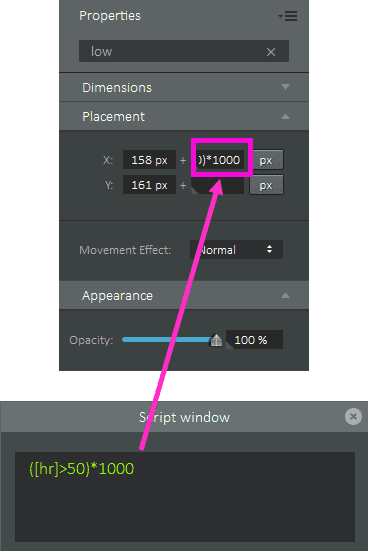• Display the animated green heart that beats slowly when the heart rate is less than or equal to 50bpm.

The initial placement of the slow heartbeat image is in the center of the watch face. When the user’s heart rate ([hr]) is less than or equal to 50bpm, the slow heartbeat image is displayed. When the heart rate is greater than 50bpm, the image is placed outside of the visible watch face.

To place the slow heartbeat image outside of the visible watch face when the heart rate is greater than 50, enough distance must be added to the placement. In this case, 1,000px is added when the heart rate is greater than 50, thus moving the placement of the image to 1,158px on the x-axis which is well outside of the visible watch face.

For the slow heartbeat image, the tag expression for the X Placement property is ([hr] > 50) * 1000. The operation in parentheses must be resolved first. The operation uses a relational operator and returns 1 (true) or 0 (false), based on the result of the operation.

If [hr] = 45,

• The relational operation ([hr]) > 50) returns 0 (false)

• X = 158px + (45 > 50) * 1000 → 158px + (0) * 1000 → 158px + 0 → 158px

• The placement is not changed and the slow heartbeat image remains visible on the watch face

If [hr] = 75,

• The relational operation ([hr]) > 50) returns 1 (true)

• X = 158px + (75 > 50) * 1000 → 158px + (1) * 1000 → 158px + 1000 → 1158px

• The placement is moved outside of the visible watch face therefore the slow heartbeat image is not displayed

If [hr] = 125,

• The relational operation ([hr]) > 50) returns 1 (true)

• X = 158px + (125 > 50) * 1000 → 158px + (1) * 1000 → 158px + 1000 → 1158px

• The placement is moved outside of the visible watch face therefore the slow heartbeat image is not displayed

The medium heartbeat image

•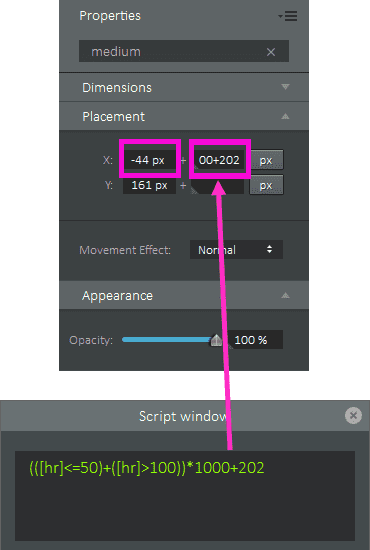• Display the animated yellow heart that beats at medium speed when the heart rate is greater than 50bpm and less than or equal to 100bpm.

The initial placement of the medium heartbeat image is outside of the visible watch face ( -44px on the x-axis). When the user’s heart rate ([hr]) is greater than 50bpm and less than or equal to 100bpm, the medium heartbeat image is displayed. When the heart rate is less than or equal to 50bpm or greater than 100bpm, the image is placed outside of the visible watch face.

To place the medium heartbeat image in the center of the watch face (the same position as the slow heartbeat image) when the heart rate is greater than 50bpm and less than or equal to 100bpm, the x-axis placement must be set to 158px. 202px is added when the heart rate conditions are met, thus moving the placement of the image to 158px on the x-axis.

For the medium heartbeat image, the tag expression for the X Placement property is (([hr] <= 50) + ([hr] > 100)) * 1000 + 202. The operation in parentheses must be resolved first. The operation uses relational operators and a logical operator that returns 1 (true) or 0 (false), based on the result of the operation.

If [hr] = 45,

• The first relational operation ([hr] <= 50) returns 1 (true) and the second relational operation ([hr] > 100) returns 0 (false)

• The logical operation (([hr] <= 50) + ([hr] > 100)) returns 1 (true) ((45 <= 50) + (45 > 100)) → ((1) + (0)) → 1

• X = -44px + ((45 <= 50) + (45 > 100)) * 1000 + 202 → -44px + ((1) + (0)) * 1000 + 202 → -44px + (1) * 1000 + 202 → -44px + 1000 + 202 → 1,158

• The placement is moved outside of the visible watch face therefore the medium heartbeat image is not displayed

If [hr] = 75,

• The first relational operation ([hr]<=50) returns 0 (false) and the second relational operation ([hr] > 100) returns 0 (false)

• The logical operation (([hr] <= 50) + ([hr] > 100)) returns 0 (false) ((75 <= 50) + (75 > 100)) → ((0) + (0)) → 0

• X = -44px + ((75 <= 50) + (75 > 100)) * 1000 + 202 → -44px + ((0) + (0)) * 1000 + 202 → -44px + (0) * 1000 + 202 → -44px + 202 → 158

• The placement is moved to the center of the watch face therefore the medium heartbeat image is displayed

If [hr] = 125,

• The first relational operation ([hr] <= 50) returns 0 (false) and the second relational operation ([hr] > 100) returns 1 (true)

• The logical operation (([hr] <= 50) + ([hr] > 100)) returns 1 (true) ((125 <= 50) + (125 > 100)) → ((0) + (1)) → 1

• X = -44px + ((125 <= 50) + (125 > 100)) * 1000 + 202 → -44px + ((0) + (1)) * 1000 + 202 → -44px + (1) * 1000 + 202 → -44px + 1000 + 202 → 1,158

• The placement is moved outside of the visible watch face therefore the medium heartbeat image is not displayed

Let’s walk through the calculation of the tag expression (([hr] <= 50) + ([hr] > 100)) * 1000 + 202 when heart rate is 75.

• Replace [hr] with the value, in this case 75: (([hr] <= 50) + ([hr] > 100)) * 1000 + 202 → ((75 <= 50) + (75 > 100)) * 1000 + 202

• The operations in parentheses must be resolved first, so resolve the relational operations (75 <= 50) and (75 > 100). Because 75 is not less than or equal to 50, the first relational operation returns 0 (false). Because 75 is not greater than 100, the second relational operation returns 0 (false): ((75 <= 50) + (75 > 100)) * 1000 + 202 → ((0) + (0)) * 1000 + 202

• Again, the operation in parentheses must be resolved first, so resolve the logical operation ((0) + (0)). In this case, + is interpreted as the OR logical operation because the operands on both sides of the operator are relational operations. (0 OR 0) returns 0 (false): ((0) + (0)) * 1000 + 202 → (0) * 1000 + 202

• Now, calculate 0 multiplied by 1000 then add 202 which results in 202. Note that * and + are interpreted as arithmetic operations because at least one of their operands is a value: (0) * 1000 + 202 → 202

• X is equal to -44px + 202 → 158px

The fast heartbeat image

•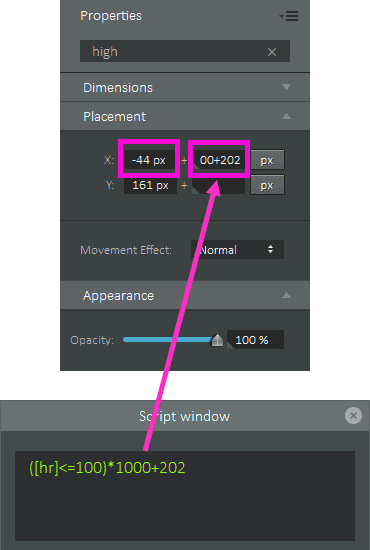• Display the animated red heart that beats fast when the heart rate is greater than 100bpm.

The initial placement of the fast heartbeat image is outside of the visible watch face ( -44px on the x-axis). When the user’s heart rate ([hr]) is greater than 100bpm, the fast heartbeat image is displayed. When the heart rate is less than or equal to 100bpm, the image is placed outside of the visible watch face.

To place the fast heartbeat image in the center of the watch face (the same position as the fast heartbeat image) when the heart rate is greater than 100bpm, the x-axis placement must be set to 158px. 202px is added when the heart rate conditions are met, thus moving the placement of the image to 158px on the x-axis.

For the fast heartbeat image, the tag expression for the X Placement property is ([hr] <= 100)) * 1000 + 202. The operation in parentheses must be resolved first. The operation uses a relational operator and returns 1 (true) or 0 (false), based on the result of the operation.

If [hr] = 45,

• The relational operation ([hr]) <= 100) returns 1 (true)

• X = -44px + (45 <= 100) * 1000 + 202 → -44px + (1) * 1000 + 202 → -44px + 1000 + 202 → 1,158px

• The placement is moved outside of the visible watch face therefore the fast heartbeat image is not displayed

If [hr] = 75,

• The relational operation ([hr]) <= 100) returns 1 (true)

• X = -44px + (75 <= 100) * 1000 + 202 → -44px + (1) * 1000 + 202 → -44px + 1000 + 202 → 1,158px

• The placement is moved outside of the visible watch face therefore the fast heartbeat image is not displayed

If [hr] = 125,

• The relational operation ([hr]) <= 100) returns 0 (false)

• X = -44px + (125 <= 100) * 1000 + 202 → -44px + (0) * 1000 + 202 → -44px + 0 + 202 → 158px

• The placement is moved to the center of the watch face therefore the fast heartbeat image is displayed